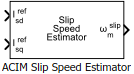# ACIM Slip Speed Estimator

Calculate slip speed of AC induction motor

Since R2020b

•Libraries:
Motor Control Blockset / Controls / Control Reference
Motor Control Blockset HDL Support / Controls / Control Reference

## Description

The ACIM Slip Speed Estimator block computes the mechanical slip speed (difference between the synchronous speed and rotor speed) of the induction motor.

The block accepts the reference values of d- and q-axis currents and outputs the computed slip speed of the induction motor.

### Equations

These equations describe the utilization of the slip speed value for field-oriented control (FOC) of the induction motor:

If we keep the rotor flux as constant and the d-axis is aligned to the rotor flux reference frame, then we can imply:

`${\lambda }_{\text{rd}}={L}_{m}{i}_{sd}$`

This block implements the preceding calculations as:

For detailed set of equations and assumptions, see Mathematical Model of Induction Motor.

where:

• ${\omega }_{\text{e_slip}}$ is the electrical slip speed of the rotor (Radians/ sec).

• ${\omega }_{\text{slip}}$ is the mechanical slip speed of the rotor (Radians/ sec).

• ${\omega }_{e}$ is the electrical speed corresponding to frequency of stator voltages (Radians/ sec).

• ${\omega }_{m}$ is the rotor mechanical speed (Radians/ sec).

• ${\omega }_{r}$ is the rotor electrical speed (Radians/ sec).

• ${L}_{\text{m}}$ is the magnetizing inductance of the motor (Henry).

• ${R}_{r}$ is the rotor resistance referred to stator (Ohms).

• ${i}_{sd}^{ref}$ and ${i}_{sq}^{ref}$ are the reference stator d- and q-axis currents (Amperes).

• ${\tau }_{\text{r}}$ is the rotor time constant (sec).

• ${\lambda }_{rd}$ is the d-axis flux linkage of the rotor (Weber).

## Ports

### Input

expand all

Reference d-axis stator current.

Data Types: `single` | `double` | `fixed point`

Reference q-axis stator current.

Data Types: `single` | `double` | `fixed point`

### Output

expand all

Mechanical slip speed of the rotor that the block computes.

Data Types: `single` | `double` | `fixed point`

## Parameters

expand all

Number of pole pairs available in the induction motor.

Rotor resistance of the induction motor in Ohms.

Inductance due to leakage flux linked to the rotor winding (in Henry).

Inductance due to the magnetizing flux (in Henry).

Saturation value for the block output (in rpm).

Unit of the input values.

Base speed (in rpm) for per-unit system.

#### Dependencies

To enable this parameter, set Input units to `Per-Unit (PU)`.

## Version History

Introduced in R2020b